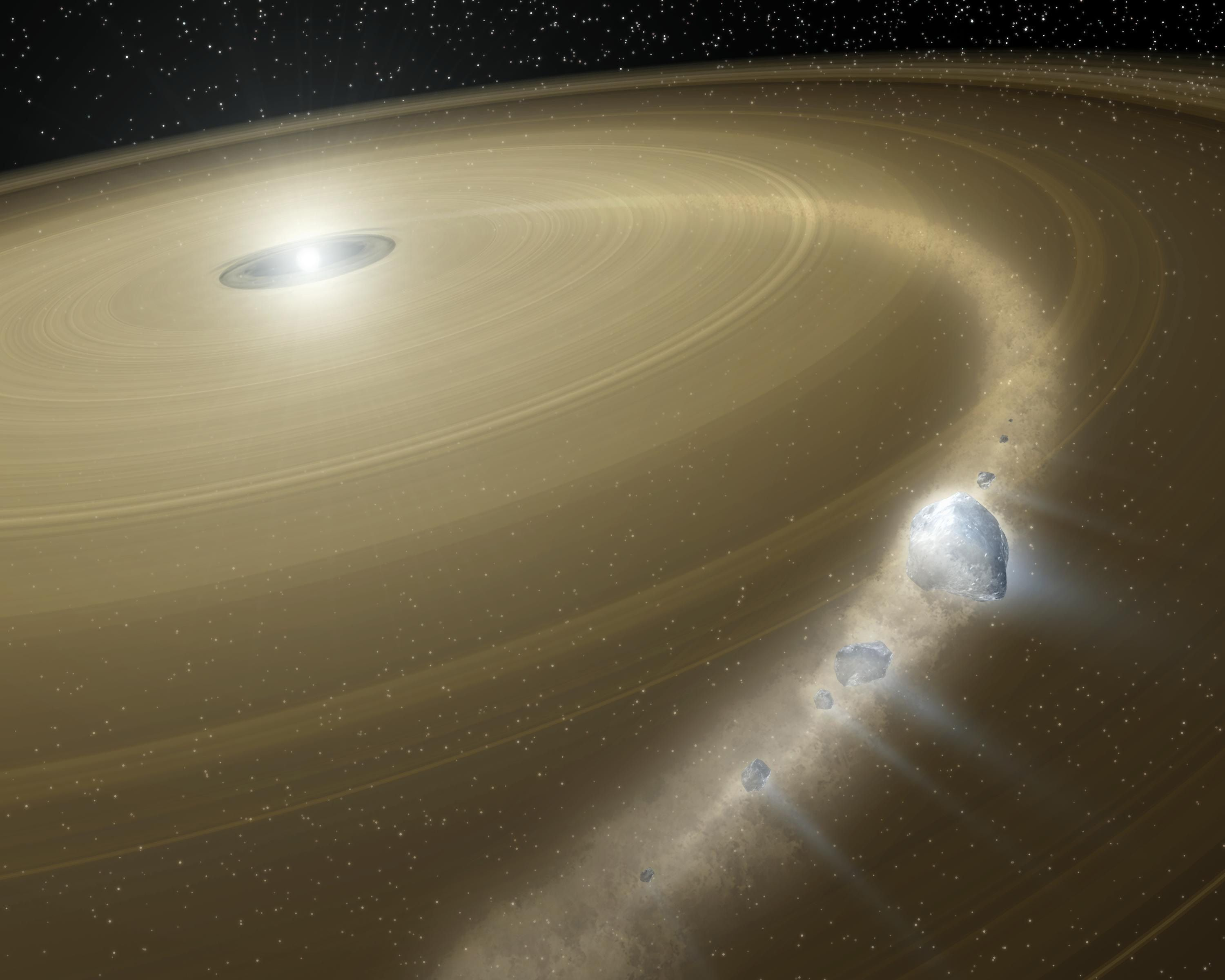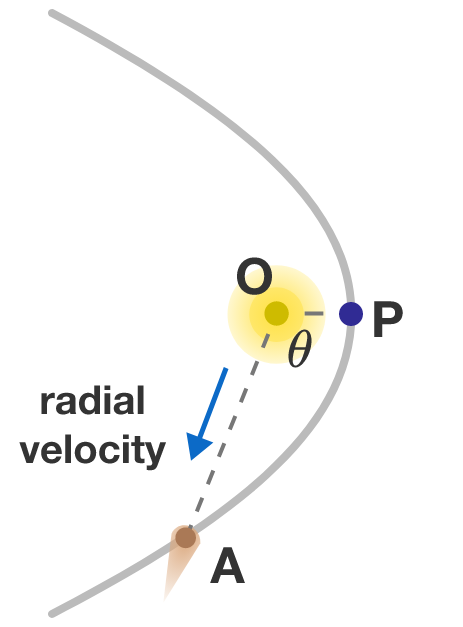# Oort Cloud Parabolic CometA comet in the Oort cloud falls towards the Sun. As it picks up speed from the Sun's gravity, it accidentally picks up a very tiny extra velocity boost from a nearby object in the solar system, so that its trajectory is now a true parabola. It eventually whips around the Sun and begins to travel back out into the far reaches of space.Let $\theta$ be the angle $\angle POA,$ where point $O$ is the center of the Sun, point $P$ is where the comet has come closest to the Sun, and point $A$ is where it is on the trajectory outward.

The radial velocity of the comet relative to the Sun is the speed in which the comet is moving away from the Sun in the direction of the line $OA$. For what angle $\theta$ is this radial velocity the greatest?


Note: Kepler orbits are commonly described by the polar equation $r(\theta)=\dfrac{a(1-e^2)}{1+e \cos(\theta)},$ where $a$ is the semi-major axis, and $e$ is the eccentricity. For a circle, $e=0;$ for an ellipse, $0 for a parabola, $e=1;$ for a hyperbola, $e>1$. Also, you may want to look up Kepler's laws and vis-viva equation.